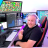# How to set a variable for use later in script

S

#### SimpleScript

##### New member
Hi folks- just started playing with thinkscript today, and came up with a script to show the ATR, 10% of ATR & 2% of ATR, with inputs for # of periods (starting at current period price, going backwards) and the type of average (Simple, Exponential, Weighted, Wilders and Hull). It seems to match the TOS provided ATR study at the last candle (which is what I want), and it takes up a lot less screen space, which is the reason for the script.

I have it all working, except when I echo the averageType, it shows a number, which I think is based on an internal conversion the interpreter is doing (Simple = 0, Exponential = 1, Weighted = 2, Wilders = 3 and Hull = 4), when I change inputs in the Customizing Study dialog.

I am trying to build the logic with a variable to display a string that is set, based on the number that averageType contains, but for the life of me I can't get the syntax right... (for example, if the average type = Simple, display "Simple" in the label, rather than the "0" that is displayed)

most recent effort was to declare the DFKTMA variable, and then set it to equal the string I want, using nested If thens, but I can't get even a basic 2 conditional to work.

am I attacking this the right way?

the commented lines are just the last iteration of about 8 different ways I have tried to set the variable DFKTMA to a string that says "Simple", rather than the "0" that is is displayed in the label.

here is the script:
Code:
``````def AvgTrueRange1;
def AvgTrueRange2;
def AvgTrueRange3;
#def DFKTMA = "Simple";

input length = 14;
input averageType = AverageType.WILDERS;
# DFKTMA=Wilders if averageType=0 then DFKTMA==Wilders else DFKTMA==test;

#if (averageType == "3", DFKTMA == “Wilders”, “Test”) ;
# def DFKTMA = "Simple" If (averageType = AverageType.SIMPLE, Else DFKTMA == "test";

AvgTrueRange1 = MovingAverage(averageType, TrueRange(high,  close,  low),  length);
AvgTrueRange2 = AvgTrueRange1 / 10;
AvgTrueRange3 = AvgTrueRange1 / 50;

AddLabel(yes, Concat(" Type of MovingAverage: ", Concat(averageType, Concat("    # of Periods: ", Concat(length, Concat("    ATR: ", Concat(AvgTrueRange1, Concat("    ATR/10%: ", Concat(AvgTrueRange2, Concat("    ATR/2%: ", AvgTrueRange3))))))))), Color.WHITE);``````

Last edited by a moderator:
S

#### SimpleScript

##### New member
got it figured out, with the help of Pete at Hahn-Tech.
I have a snapshot, but here's the code for anyone interested:

Code:
``````# dec DFKTMA == "SimpleScript";
# by DFK & Associates.  Copyright 2020. This code is distributed freely without restriction.  Use in any way absolves author of any and all liability.
def AvgTrueRange;
def AvgTrueRange10;
def AvgTrueRange2;

input length = 14;
input AverageType = AverageType.WILDERS;

AvgTrueRange = MovingAverage(AverageType, TrueRange(high,  close,  low),  length);
AvgTrueRange10 = AvgTrueRange / 10;
AvgTrueRange2 = AvgTrueRange / 50;

AddLabel(yes, Concat(" Type of Moving Average:  ", Concat((if AverageType == AverageType.Wilders then "Wilders" else if AverageType == AverageType.Simple then "Simple"  else if AverageType == AverageType.Hull then "Hull"  else if AverageType == AverageType.Exponential then "Exponential" else "Weighted"), Concat("    # of Periods: ", Concat(length, Concat("    ATR: ", Concat(AvgTrueRange, Concat("    10% ATR: ", Concat(AvgTrueRange10, Concat("    2% ATR: ", AvgTrueRange2))))))))), Color.WHITE);``````

Last edited by a moderator:Set Position Exit to Low/High before Entry Bar? Questions 2